NEET  >  Test: Potential Energy

# Test: Potential Energy

Test Description

## 20 Questions MCQ Test Physics Class 11 | Test: Potential Energy

Test: Potential Energy for NEET 2022 is part of Physics Class 11 preparation. The Test: Potential Energy questions and answers have been prepared according to the NEET exam syllabus.The Test: Potential Energy MCQs are made for NEET 2022 Exam. Find important definitions, questions, notes, meanings, examples, exercises, MCQs and online tests for Test: Potential Energy below.
Solutions of Test: Potential Energy questions in English are available as part of our Physics Class 11 for NEET & Test: Potential Energy solutions in Hindi for Physics Class 11 course. Download more important topics, notes, lectures and mock test series for NEET Exam by signing up for free. Attempt Test: Potential Energy | 20 questions in 20 minutes | Mock test for NEET preparation | Free important questions MCQ to study Physics Class 11 for NEET Exam | Download free PDF with solutions
 1 Crore+ students have signed up on EduRev. Have you?
Test: Potential Energy - Question 1

### How much work is done by a man weighing 80 kgf in raising a stone of weight 20 kgf to the top of a building of height 40 m? (g = 9.8 m/s2)

Detailed Solution for Test: Potential Energy - Question 1

Total mass=80+20=100
w=mgh(where m=100, g=9.8, h=40m)
w=100×9.8×40
w=39200J
work done by man weighing 80 kg raising a stone of 20kgf to the top of building of Height is 40m is 39,200J

Test: Potential Energy - Question 2

### The potential energy of a body at height h is mgh. Then its kinetic energy just before hitting the ground is

Detailed Solution for Test: Potential Energy - Question 2

From the law of conservation of mechanical energy, the potential energy of a body at height h is converted into kinetic energy when it falls down. So, the magnitude of kinetic energy just before hitting the ground is equal to the potential energy at height h which is mgh.

Test: Potential Energy - Question 3

### When does the potential energy of a spring increase?

Detailed Solution for Test: Potential Energy - Question 3

Potential energy of a spring is proportional to the square of the difference of the springs length and its original length, hence whether it is compressed or stretched the potential the potential energy will eventually increase only.

Test: Potential Energy - Question 4

In the given figure, what is the work done by the spring force?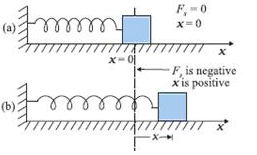Detailed Solution for Test: Potential Energy - Question 4

We know that for a spring with spring constant k, work done by the spring when a compression or expansion is done of some length say x is ½ kx2
Also as the work done is in the opposite direction to that of force applied, we get the sign of the force to be negative.

Test: Potential Energy - Question 5

Which of the following force is non-conservative

Detailed Solution for Test: Potential Energy - Question 5

Force between two stationary mass is gravitational force which is conservative. between two charge is electrostatic. muscular force is non conservative because we did work it lost or appear in form of heat and we need to eat food to gain energy for work done.

Test: Potential Energy - Question 6

A body dropped from height H reaches the ground with a speed of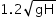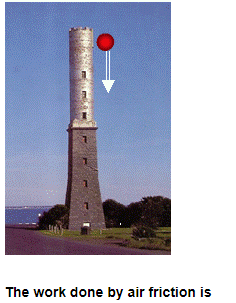Detailed Solution for Test: Potential Energy - Question 6

The forces acting on the body are the force of gravity and the air friction. By work energy theorem, the total work done on the body is
W=1/2m(1.2√gH)2−0=0.72mgH
The work done by the force of gravity is mgH.
. Hence, the work done by the air friction is,
=0.72mgH-mgH
=00.28mgH.

Test: Potential Energy - Question 7

You are in a lift moving from the 3rd floor to the 12th floor, through a height H. If the elevator moves at a constant speed without stopping, what is the work performed on you by the elevator? Take your body mass as M.

Detailed Solution for Test: Potential Energy - Question 7

According to Newton's third law of motion, the force exerted on the floor by the person is equal and opposite the force exerted on the person by the floor.
According to the second law of Newton's law of motion, the net force exerted on the person is equal to the person’s mass times the person’s acceleration.
The net force exerted on the person = Force exerted by the floor+ Force exerted by gravity.
If the elevator goes up with acceleration g, the same is the person inside the elevator.
The work done on the man by the floor is the sum of the change in potential energy and kinetic
energy.
i.e W = Δ P.E + Δ K.E
But the elevator moves at a constant speed, so K.E =0.
W = Δ P.E
The work performed on you by the elevator is force times displacement = -Mgh
Negative sign shows it is moving upwards, i.e., against g.

Test: Potential Energy - Question 8

An astronomer has total weight of 80 kg. On reaching moon, he climbs up 10 m to enter his aircraft by the ladder. His gravitational potential energy is increased by 1000 J. The value of the gravitational acceleration on the moon is

Test: Potential Energy - Question 9

Out of a pair of identical springs of spring constants 240 N/m, one is compressed by 10 cm and other is stretched by 10 cm. The difference in P.E. stored in the two springs is:

Detailed Solution for Test: Potential Energy - Question 9

P.E. stored in a spring = 1/2Kx2
where x is the extension or compression. In both cases, work is done on the spring.
∴ For the same x, the same potential energy will be stored in the springs.
∴ The difference between the energies is zero.

Test: Potential Energy - Question 10

What is the dimension of k/m where k is the force constant and m is the mass of the oscillating object?

Detailed Solution for Test: Potential Energy - Question 10

F=k x (compression in string).
X=L.
a=LT-2.
F=ma.
ma= k L.
LT-2/L=k/m.
T-2=k/m.

Test: Potential Energy - Question 11

A ball of mass 2 kg is raised to the height of 60 m and then dropped from the top. In this case, the quantity which has different magnitude from the other given quantities is

Detailed Solution for Test: Potential Energy - Question 11

We know that the total work done by gravity is always equal to the gravitational force in magnitude as it is a conservative force and as because no other non conservative force acts upon the body we can say the mechanical energy remains conserved. Thus we get the potential energy at top is exactly equal to KE just before the ball hitting the ground.

Test: Potential Energy - Question 12

A hawk of mass 10 kg dives to catch a mouse. During dive it loses 500 J of its potential energy. The height from where it begins its dive is (take g = 10 m/s2)

Detailed Solution for Test: Potential Energy - Question 12

Change in GPE (Gravitational Potential energy) = mass x gravity x change in height
500 = 10 x 10 x change in height

500 / 100 = change in height

So, height from where it begins its dive = 5 m

Test: Potential Energy - Question 13

A vehicle of 50 quintal climbs up a hill of 10 m. The potential energy gained by it is (take g = 10 m/s2)

Detailed Solution for Test: Potential Energy - Question 13

mass = 50 x 100 = 5000 kg, g = 10 m/s2,  h = 10 m
Potential energy gained = mgh = 5000 x 10 x 10 = 5 x 105 J

Test: Potential Energy - Question 14

Select the odd one out

Detailed Solution for Test: Potential Energy - Question 14

In A stretched bow. Compressed gas, compressed or elongated spring there is elastic potential energy but the lifted weight there is gravitational force, so the odd one here is lifted weight.

Test: Potential Energy - Question 15

You are in a lift moving from the 3rd floor to the 12th floor, having a height difference of H = 40 m. Your mass is M = 80 kg and the acceleration due to gravity is g = 10 m/s2. If the elevator moves at a constant speed without stopping, what is the total work performed by all of the forces together (the net force) acting on you?

Detailed Solution for Test: Potential Energy - Question 15

As the final velocity after the action of all forces is 0, therefore the person has 0 net acceleration.

And, as we know that

Fnet = m.anet

Therefore, the net force is 0 and hence, the net work done is also 0.

Test: Potential Energy - Question 16

An elastic spring of force constant k is compressed by an amount x. Then its potential energy will be

Detailed Solution for Test: Potential Energy - Question 16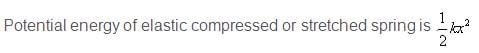Test: Potential Energy - Question 17

A plot of potential energy and kinetic energy versus displacement x for a block attached to a spring obeying Hooke’s law is

Detailed Solution for Test: Potential Energy - Question 17

The graphs are parabolas as the potential and kinetic energy vary as x2.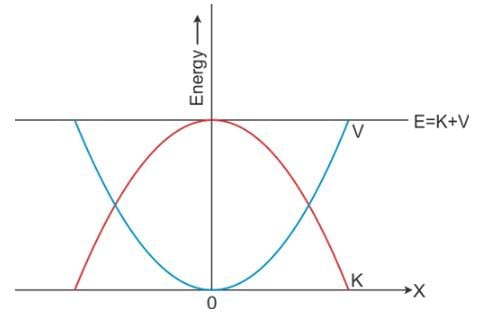Test: Potential Energy - Question 18

The principle of conservation of energy is represented by

Detailed Solution for Test: Potential Energy - Question 18

The law of conservation of energy states that: the total mechanical energy of a system remains unchanged (constant) in any isolated system of objects that interact only through conservative forces. In other words, it states that: the energy may neither be created nor destroyed, but transferred from a form of energy to another. The total mechanical energy, E, is defined as:[ E = K + U] Where K is the total kinetic energy and U is the total potential energy of the system.

The conservation law of energy is , therefore, [Ei = Ef ],Where Ei and Ef are the initial and final energies, respectively. That implies to Ki + Ui = Kf + Uf

Test: Potential Energy - Question 19

Which of the following is not a kind of potential energy?

Detailed Solution for Test: Potential Energy - Question 19

Potential Energy is any type of stored energy. It can be chemical, nuclear, gravitational, or mechanical. Kinetic Energy is found in movement. Nuclear power plants change the nuclear potential energy of uranium or plutonium into electricity too.

Test: Potential Energy - Question 20

For a varying force work done can be expressed as a

Detailed Solution for Test: Potential Energy - Question 20

For a variable force, the work done can be expressed as a definite integral of force over displacement for any system.In case of a variable force, the is calculated with the help of integration. For example, in the case of a spring, the force acting upon any object attached to a horizontal spring can be given as Fs = -kx, where k is the spring constant and x is the displacement of the object attached. We can see that this force is proportional to the displacement of the object from the equilibrium position, hence the force acting at each instant during the compression and extension of the spring will be different. Thus, the infinitesimally small contributions of work done during each instant are to be counted in order to calculate the total work done.

## Physics Class 11

126 videos|449 docs|210 tests
 Use Code STAYHOME200 and get INR 200 additional OFF Use Coupon Code
Information about Test: Potential Energy Page
In this test you can find the Exam questions for Test: Potential Energy solved & explained in the simplest way possible. Besides giving Questions and answers for Test: Potential Energy, EduRev gives you an ample number of Online tests for practice

## Physics Class 11

126 videos|449 docs|210 tests

### How to Prepare for NEET

Read our guide to prepare for NEET which is created by Toppers & the best Teachers